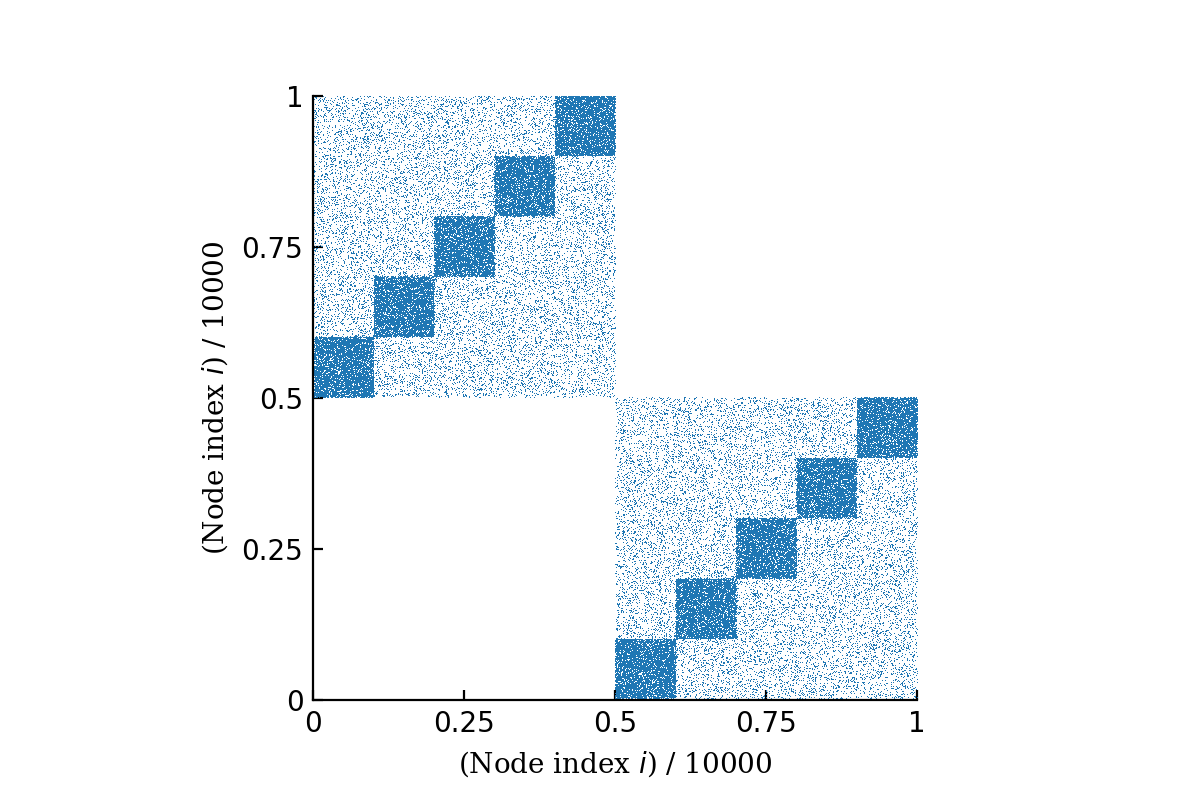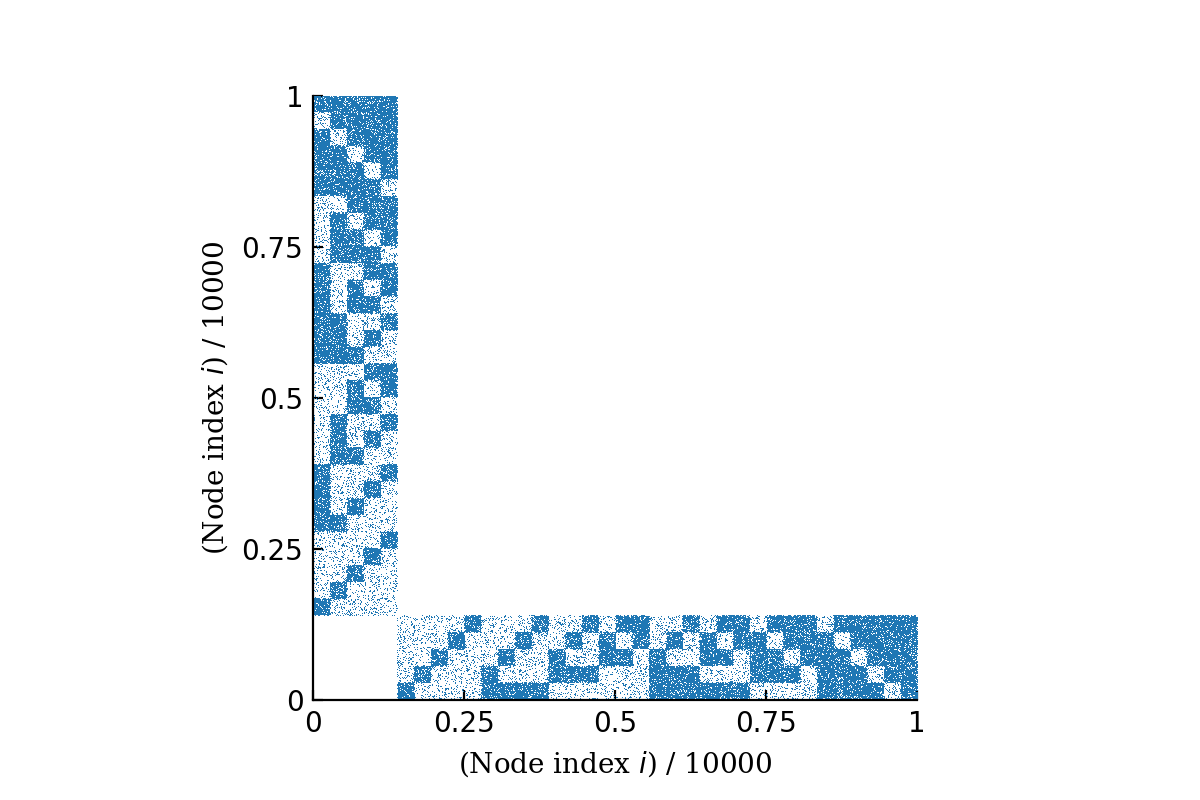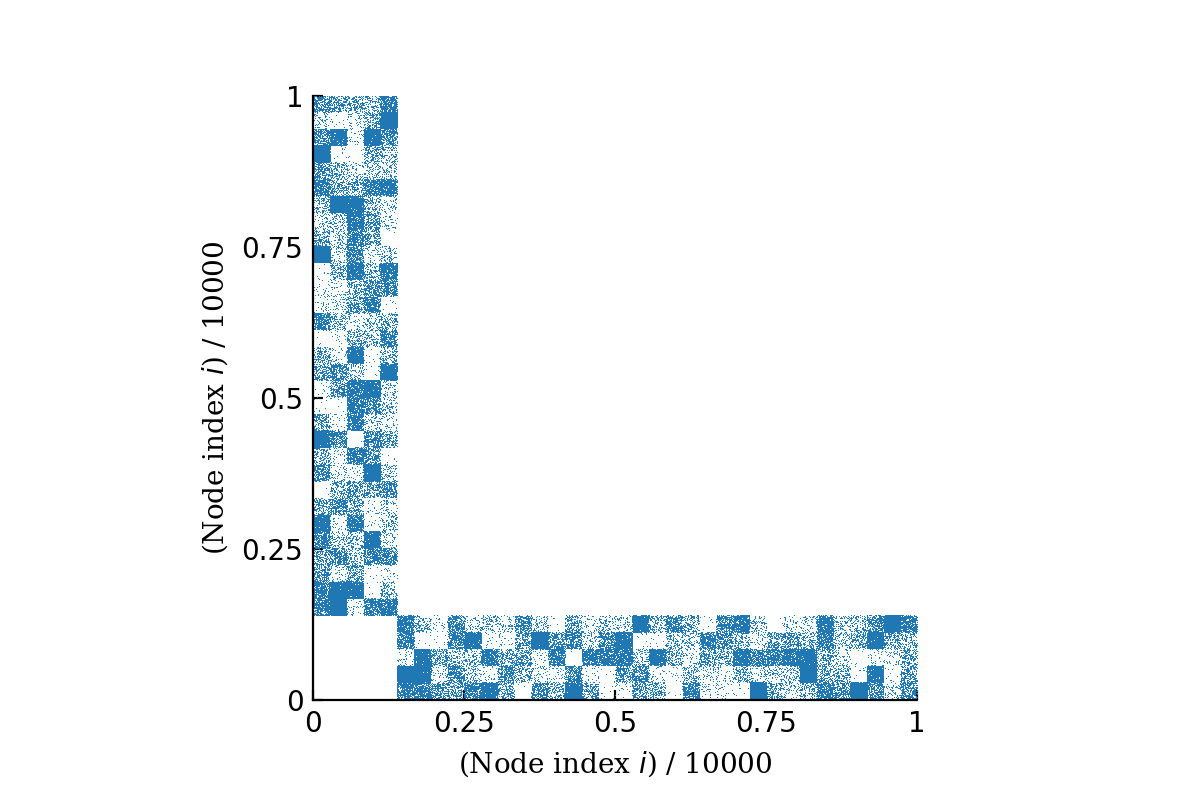# Generate synthetic networks¶

It is necessary to benchmark our algorithm via synthetic networks, where we have perfect confidence on the model parameters. Here we can control the nodes’ block membership $$b$$, the edge count matrix $$e_{rs}$$, and the degree distribution $$d$$. Once we specified these 3 parameters, we call Graph-tool’s generate_sbm function to generate a graph-tool graph instance, and then use that instance as an input for our biSBM.optimalks.OptimalKs class.

## Edge count matrix $$e_{rs}$$¶

### Easy cases¶

The easy cases are bipartite version of the planted partition models. It is a $$(K_a, K_b)$$-bipartite structure with $$e_{rs} = p c / K + (1 - p)(1 - c)/K(K-1)$$, where $$K_a = K_b = K$$ and $$p = c_{out}/c_{in}$$ is a parameter that controls how crisp the structure is. When $$p = 0$$, we expect no connections at all between nodes of different groups, i.e., $$e_{rs} = E \delta_{rs}/ K$$.A easy case with $$\langle k\rangle = 10$$ and $$p=0.1$$, planted as $$(K_a, K_b) = (5, 5)$$ equally-sized communities.

### Hard cases¶

The hard cases are planted networks where $$K_a \neq K_b$$, but the edge count matrix is still designed in a way that allow the control of network structural strength.A hard case with $$\langle k\rangle = 10$$ and $$p=0.1$$, planted as $$(K_a, K_b) = (5, 31)$$ equally-sized communities.

### Even harder cases¶

A even harder case is simply a random draw out of the ensemble of edge count matrices where we only fix $$K_a$$ and $$K_b$$.A harder case with $$\langle k\rangle = 10$$, planted as $$(K_a, K_b) = (5, 31)$$ equally-sized communities. This is the strongest structure out of $$10^6$$ random $$e_{rs}$$ draws.